# ML Wiki

## Box Plot

Box Plot

General idea: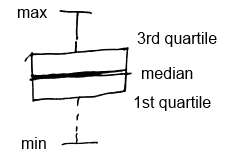• IQR = Q3 - Q1 - the length of the box
• whiskers (fences) capture data outside of the box

boxplot(..., range=0, ...)
boxplot(..., horizontal=T, ...) // horizontal boxplot


range=0 means that it will show usual box plot.

### Modified Box Plot

Modified box plot can be used to show Outliers

• IQR (Inter Quartile Range) - difference between 3rd and 1st quartile
• Inner fences - the values that are 1.5 times the IQR beyond the 1st and 3rd quartile
• Lower inner fence = 1st quartile - (1.5 x IQR)
• Upper inner fence = 3rd quartile + (1.5 x IQR)
• observations beyond the whiskers (fences) are outliers and marked with dots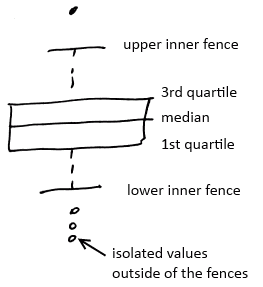In R

• by default boxplot shows modified box plot
• IQR(data) shows the IQR

## Bivariate Analysis

We can calculate all 5 number values for all quantitative variables associated with a specific category.

• And for each category get a box plot
• With box plots, we also can see how two values interact
•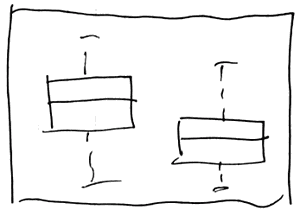### R

boxplot(d$a ~ as.factor(d$f))
• it will show separate boxplot of values in $a$ for each values of $f$
•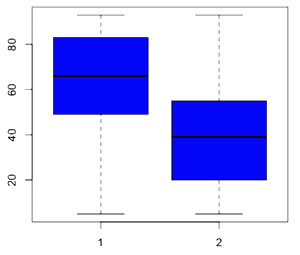boxplot(d$a ~ as.factor(d$f), col=c("blue","orange"), names=c("yes","no"), varwidth=T)

• if we want to show how much data is there for each factor,
• we can make the with of the boxes proportional to the volume of data
• using varwidth=T
•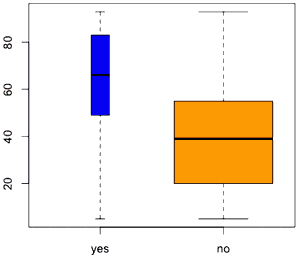## Box Plot with Other Plots

Box plots are nice to combine with other plots

• for example, with a Scatter Plot
•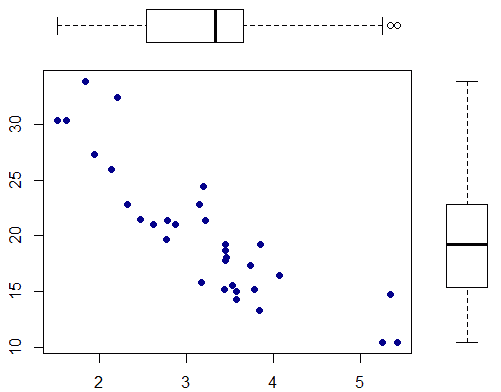• This is the R snipped to produce this figure